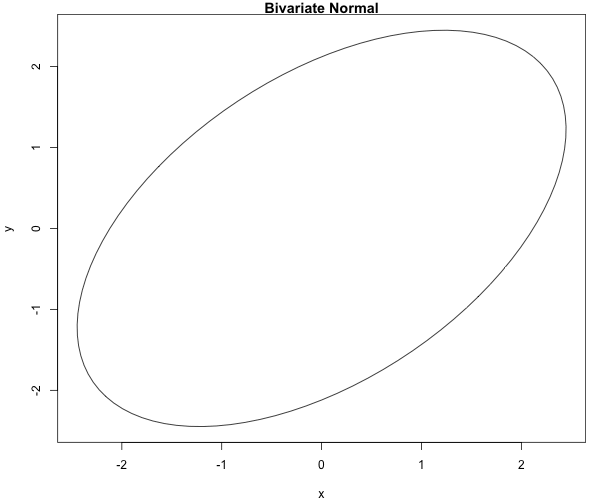# Gibbs Sampler Examples¶

## Bivariate Normal¶

Suppose we want to simulate from a centered bivariate normal ($$\mu=0$$) with correlation $$\rho$$ and variances both 1 and we know that $$X|y \sim \mathcal{N}(\rho y,1-\rho^2)$$ (A proof can be found here ):

##########################################Function######
gibbsNimate <- function(NSimul=50, rho=0.5, x0=1, y0=1)
{
##NSimul=Number of simulations
##rho is the correlation
##x0 and y0 are the starting values
## plot the 95% contour of the theoretical normal
##
plot(ellipse(rho), type="l",
main="Bivariate Normal", col="green")
dev.hold()
## initialization
x <- rep(0, NSimul)
y <- rep(0, NSimul)
x=x0
y=y0
## Gibbs sampler and plot points

for(b in 2:NSimul) {
plot(ellipse(rho), type="l",
main="Bivariate Normal")
points(x[1:(b-1)], y[1:(b-1)])
dev.hold()
## sample the x direction conditional on y
x[b] <- rnorm(1, rho*y[b-1], sqrt(1-rho^2))
lines(c(x[b-1], x[b]), c(y[b-1], y[b-1]))
## sample the y direction conditional on x
y[b] <- rnorm(1, rho*x[b], sqrt(1-rho^2))
lines(c(x[b], x[b]), c(y[b-1], y[b]))
ani.pause()
points(x[b], y[b],col="red")
}
## return the draws
return(cbind(x,y))
}


If we run this function with a correlation of 0.5.

#################
require(ellipse)
require(animation)
#oopt = ani.options(interval = 2)
#gibbsNimate(50,rho=0.5)


We obtain the 50 first steps: